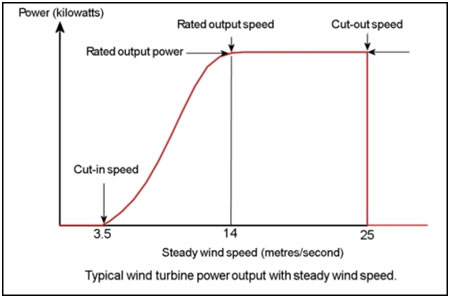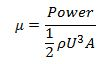### Introduction

The figure below shows a sketch on how the power output from a wind turbine varies with wind speed.Figure: Power Output Variation

Cut-in Speed: At very low wind speeds, there is insufficient torque exerted by the wind on the turbine blades to make them rotate. However, as the speed increases, the wind turbine will begin to rotate and generate electrical power. The speed at which the turbine first starts to rotate and generate power is called the cut-in speed and is typically between 3 and 4 metres per second.

Rated output power and rated output wind speed: As the wind speed rises above the cut-in speed, the level of electrical output power rises rapidly as shown. However, typically somewhere between 12 and 17 metres per second, the power output reaches the limit that the electrical generator is capable of. This limit to the generator output is called rated power output and the wind speed at which it is reached is called the rated output wind speed. At higher wind speeds, the design of the turbine is arranged to limit the power to this maximum level and there is no further rise in the output power. How this is done varies from design to design but typically with large turbines, it is done by adjusting the blade angles so as to keep the power at the constant level.

Cut-out speed: As the speed increases above the rate output wind speed, the forces on the turbine structure continue to rise and, at some point, there is a risk of damage to the rotor. As a result, a braking system is employed to bring the rotor to a standstill. This is called the cut-out speed and is usually around 25 metres per second.

Wind turbine efficiency or power coefficient: The available power in a stream of wind of the same cross-sectional area as the wind turbine can easily be shown to be:Where the wind speed U is in metres per second, the density ρ is in kilograms per cubic metre and the A is the corss-sectional area in square meters then the available power is in watts. The efficiency, μ, or, as it is more commonly called, the power coefficient, cp, of the wind turbine is simply defined as the actual power delivered divided by the available power:• N/A